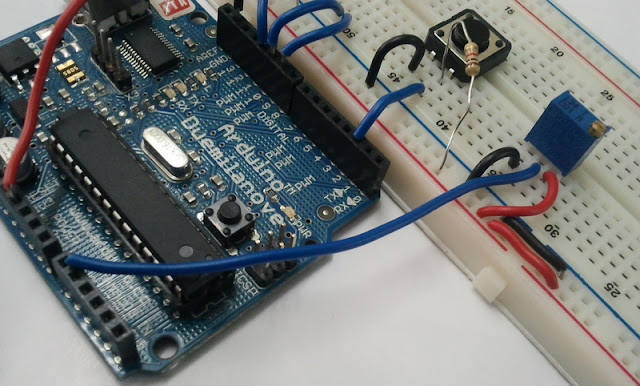## Wednesday, May 16, 2012

### Simple Labs' Quick Start Kit for Arduino - Trimpot / Potentiometer Interfacing - How To?

Trimpot
Trimpots are used for getting variable / adjustable user inputs. Common example of a trimpot is the volume knob of your stereo player, Tuning knob of the radio, etc.

A Trimpot aka variable resistor has three pins and an adjustment screw. A Trimpot acts as a potential divider and gives an output voltage on the 2nd pin. This output voltage is in-between the voltages supplied to the 1st and 3rd pins. The output voltage can be varied by adjusting the screw. The 1st and 3rd pins are connected to SUPPLY / GROUND and the middle pin is connected to Analog Input 0 on the arduino using a wire.

Connect the trimpot as shown in the images belowThe Trimpot - See the markings for pinsPlace the trimpot as shownConnect a wire between pin 1 of the trimpot and the '+'ve terminalConnect a wire between pin 3 of the trimpot and the '-'ve terminalConnect a wire between pin 2 of the trimpot and the Analog In 0 of the ArduinoHere is the connection going to the Analog In 0
The Analog Input pins on the Arduino let you connect sensors and other analog devices like trimpots that product a voltage output in the range of 0-5 volts. The Analog input on Arduino is of 10-bit resolution. 10-bit resolution means that the Voltage range of 0-5 Volts is represented in 1024 steps from 0-1023. So you would be reading an input value in the range of 0-1023 where 1023 would correspond to 5 Volts

Arduino[the IDE!] comes with a serial library that can be used to transmit data serially to a computer. We shall make use of this library to transmit our trimpot value to a Computer every 1 second. You can open the 'Serial Monitor' in the Arduino IDE to view these values. You can open the 'Serial Monitor' by going to the 'Tools' menu. When using the 'Serial Monitor' ensure that the baud rate selected is the same as the one used in the program.

Try the following program and see the values you get by varying the trimpot [ simple_trimpot.ino]

```/*
A Simple Program to display the value read from the trimpot onto the Serial Monitor
*/

int intensity = 0;

void setup() {
Serial.begin(9600);
}

void loop() {
intensity = analogRead(0);
Serial.print("Current Value:");
Serial.print("   ");
Serial.println(intensity);
}
```
If you notice, you will see values from 0-1023. Now we are going to use the trimpot as part of our previous experiment. We will use the button to select the active color of the RGB LED and then use the trimpot to set the intensity of that color. Remember that this would involve scaling 0-1023 to 0-255 (or divide by 4!)

Try the following program [RGB_button_trimpot.ino]
```/*
Pressing the Button changes the current active color of the RGB LED and varying the trimpot changes the intensity of the color
*/

int intensity = 0, pin = 9;

void setup() {
pinMode(2,INPUT);
Serial.begin(9600);
}

void loop() {
if(digitalRead(2)==0) // Switch being pressed
{
if(pin<11)
pin++;
else
pin=9;
analogWrite(9,0);
analogWrite(10,0);
analogWrite(11,0);
while(digitalRead(2)==0);
delay(100);
}
intensity = analogRead(0)/4; // Scaling the input resolution to match with our output resolution.
analogWrite(pin,intensity);
Serial.print(pin);
Serial.print("   ");
Serial.println(intensity);
}

```
Heres the final setup and the output!